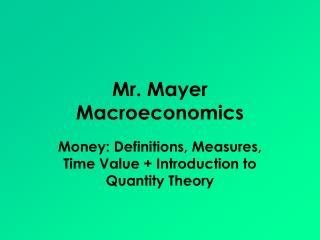# Mr. Mayer Macroeconomics - PowerPoint PPT PresentationDownload PresentationMr. Mayer Macroeconomics

Mr. Mayer Macroeconomics
Download Presentation## Mr. Mayer Macroeconomics

- - - - - - - - - - - - - - - - - - - - - - - - - - - E N D - - - - - - - - - - - - - - - - - - - - - - - - - - -
##### Presentation Transcript

1. Mr. Mayer Macroeconomics Money: Definitions, Measures, Time Value + Introduction to Quantity Theory

2. Money Defined • Money is anything that can be used as: • A medium of exchange • A store of value • A unit of account / Standard of Value • Money works best when it meets these criteria: • Portable • Durable • Divisible • Acceptable • Stable

3. Money Facts: • What backs the dollar and makes it valuable? • Gold? • NO! The dollar is legal tender because the government says it’s money and people willingly accept it. The Dollar is backed by FAITH. • This is referred to as an inconvertible fiat standard.

4. The Supply of Money • In the United States, the Federal Reserve System is the sole issuer of currency. • This means the Fed has monopoly control over the money supply. • There are two important measures of the Money Supply today. • M1 • M2

5. M1 • M1 serves primarily as a medium of exchange. It includes: • Currency and Coin • Demand Deposits

6. M2 • M2 serves as a store of value. It includes: • The M1 • Time Deposits • Money Market Mutual Funds • Overnight Eurodollars Certificates of Deposit Savings Accounts

7. M1 & M2 • As we go from M1 to M2 • The measure becomeslarger • Money becomes less liquid • As we go from M2 to M1 • Themeasurebecomes smaller • Money becomesmoreliquid

8. Time Value of Money • Is a dollar today worth more than a dollar tomorrow? • YES • Why? • Opportunity cost & Inflation • This is the reason for charging and paying interest ???

9. Time Value of Money • Let v = future value of \$ p = present value of \$ r = real interest rate (nominal rate – inflation rate) expressed as a decimal n = years k = number of times interest is credited per year • The Simple Interest Formula v = ( 1 + r )n*p • The Compound Interest Formula v = ( 1 + r/k )nk* p

10. Time Value of MoneyIllustrated • Assume that inflation is expected to be 3% and that the nominal interest rate on simple interest savings is 1%. Calculate the future value of \$1 after 1 year. • Step 1: Calculate the real interest rate r% = i% - p% r% = 1% - 3% = -2% or -.02 • Step 2: Use the simple interest formula to calculate the future value of \$1 v = ( 1 + r )n*p v = ( 1 + (-.02))1 * \$1 v = (.98) * \$1 v = \$0.98

11. Time Value of MoneyIllustrated • Assume that inflation is still expected to be 3% but that the nominal interest rate on simple interest savings is 4%. Calculate the future value of \$1 after 1 year. • Step 1: Calculate the real interest rate r% = i% - p% r% = 4% - 3% = 1% or .01 • Step 2: Use the simple interest formula to calculate the future value of \$1 v = ( 1 + r )n*p v = ( 1 + .01)1 * \$1 v = \$1.01

12. Time Value of MoneyFUN!!! • Assume that annual inflation is expected to be 2.5% and that the annual nominal interest rate on a 10 year certificate of deposit is 5% compounded monthly. Calculate the future value of \$1,000 after 10 years. • Step 1: Calculate the real interest rate r% = i% - p% r% = 5% - 2.5% = 2.5% or .025 • Step 2: Use the compound interest formula to calculate the future value of \$1,000 v = ( 1 + r/k )nk*p v = ( 1 + .025/12)10*12 *\$1,000 v = ( 1 + 0.002083)120 *\$1,000 v = \$1,283.69

13. Relating Money to GDP • Economist, Irving Fisher postulated that : Nominal GDP = The Money Supply * Money’s Velocity

14. The Monetary Equation of Exchange • MV = PQ • M = money supply (M1 or M2) • V = money’s velocity (M1 or M2) • P = price level (PL on the AS/AD diagram) • Q = real GDP ( sometimes labeled Y on the AS/AD diagram) • P*Q or PQ = Nominal GDP

15. The Monetary Equation of Exchange • MV=PQ • M1=\$2 trillion • V of M1 = 7 • PQ = \$14 trillion LRAS SRAS PL P AD QF GDPR